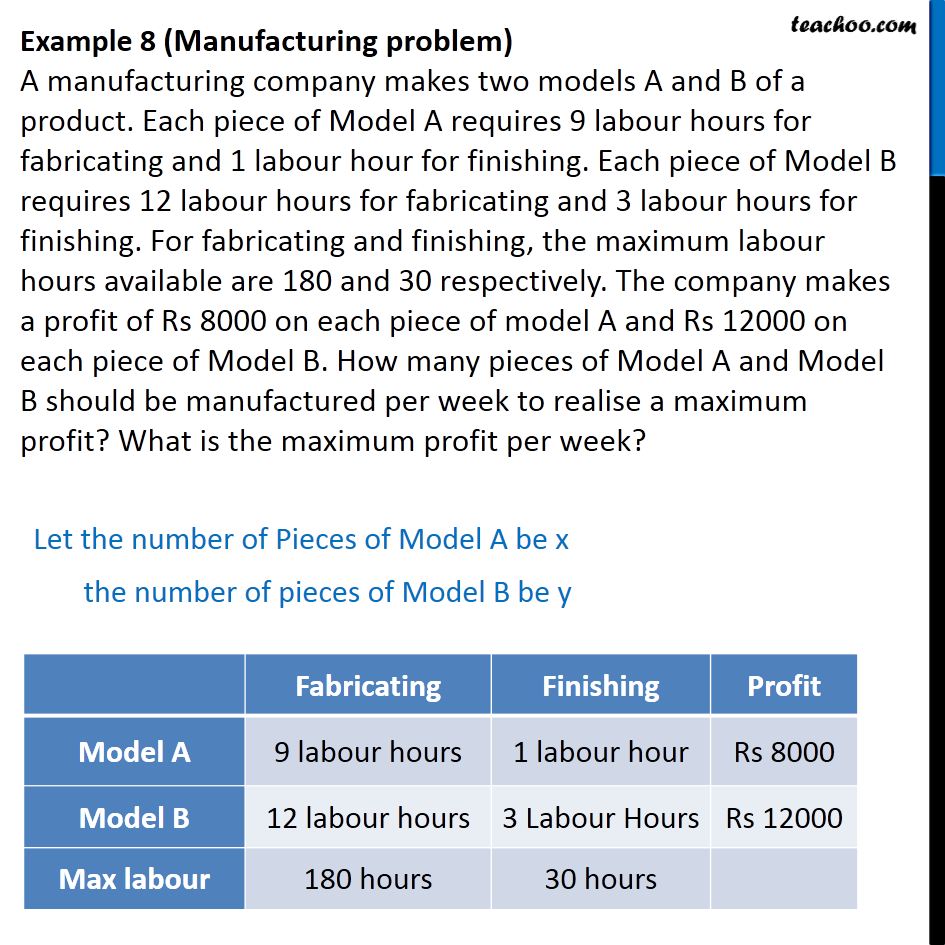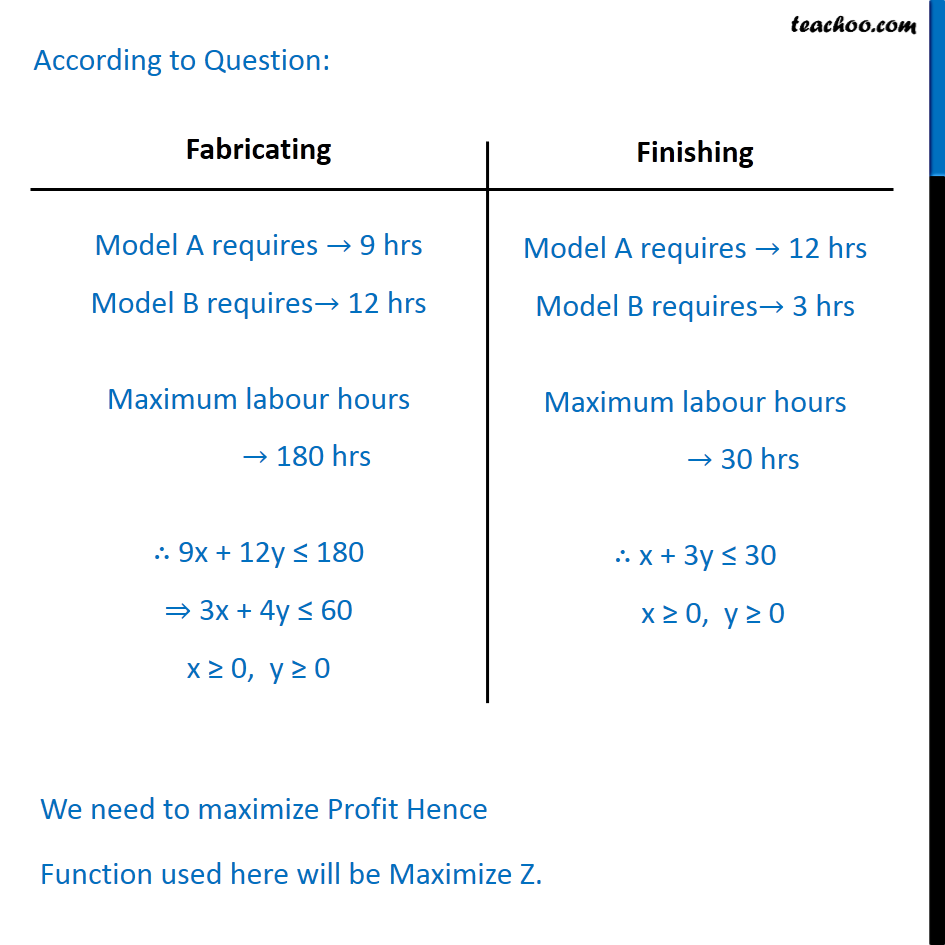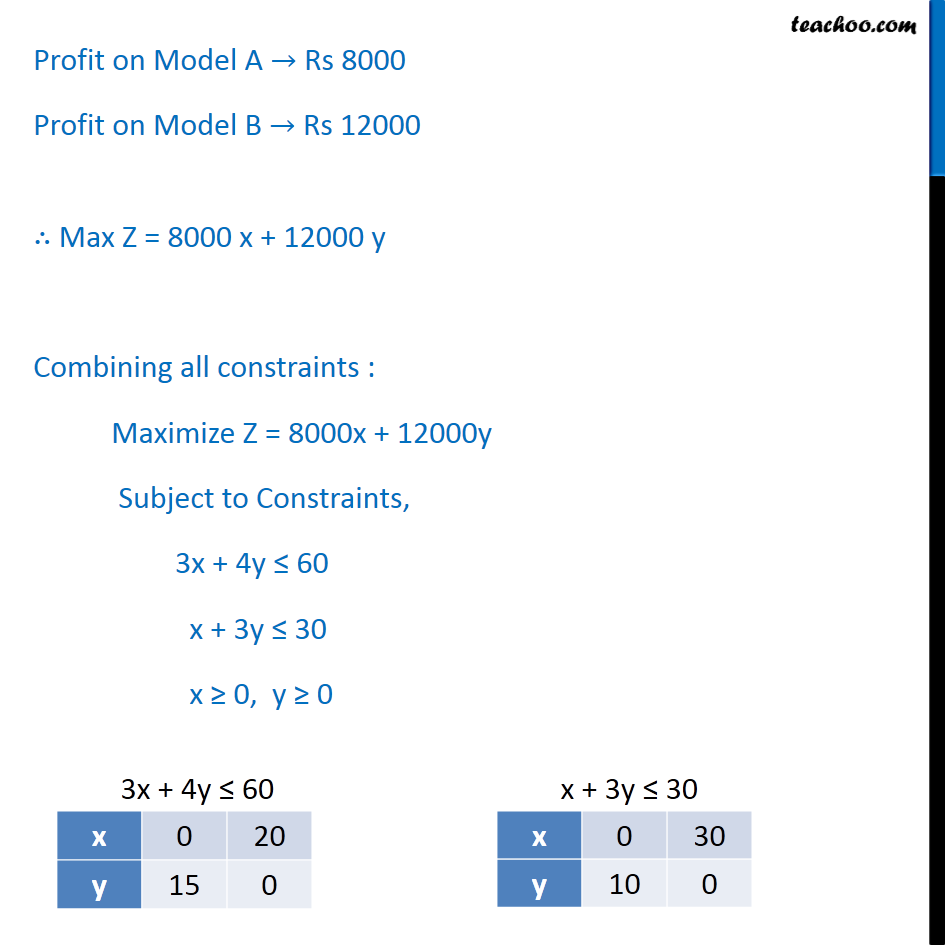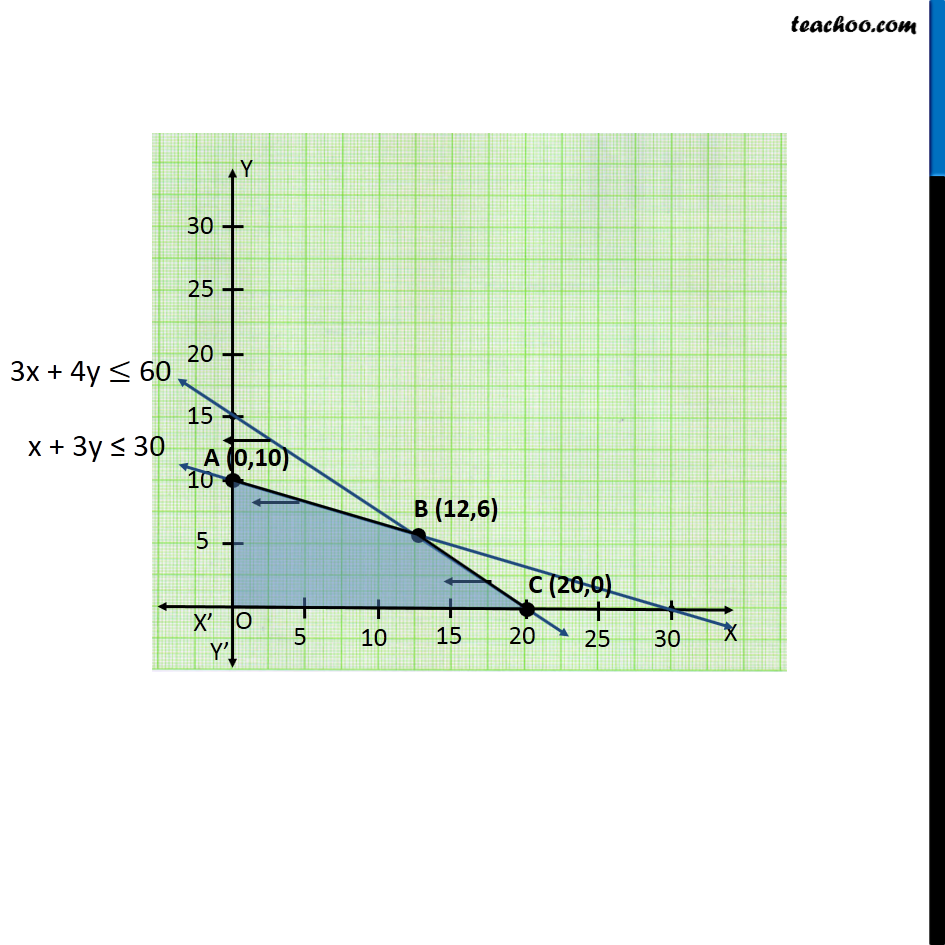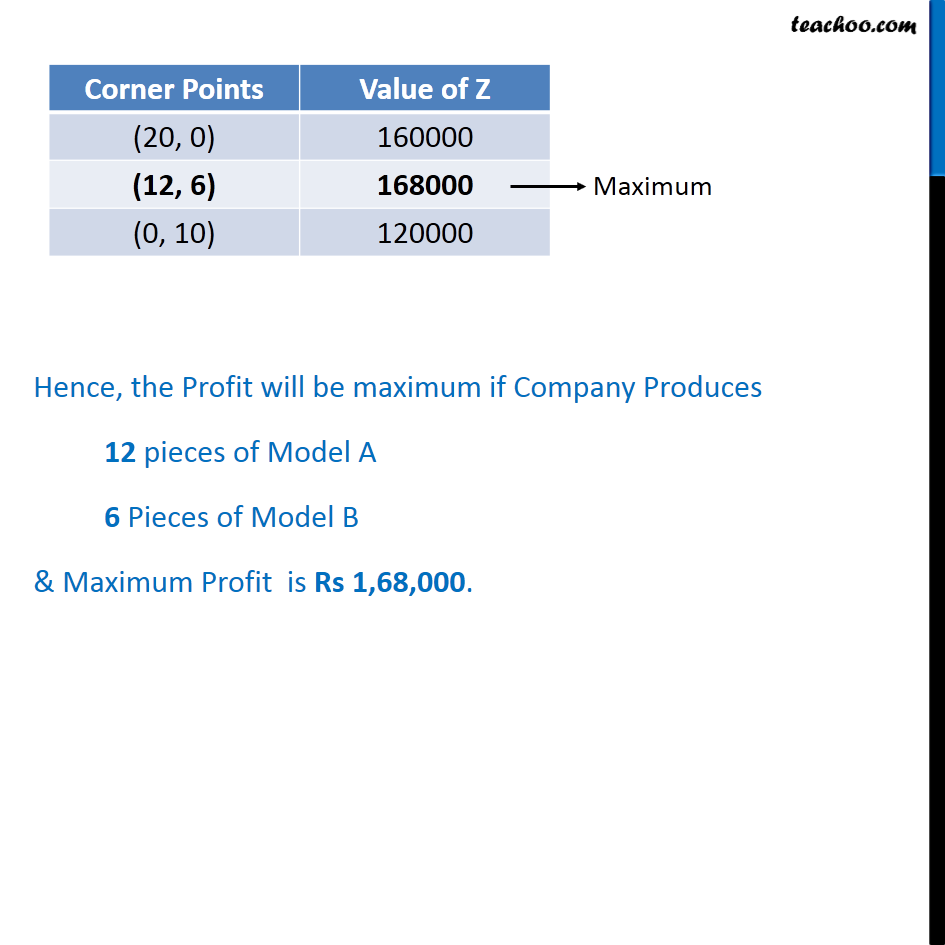1. Chapter 12 Class 12 Linear Programming
2. Serial order wise
3. Examples

Transcript

Example 8 (Manufacturing problem) A manufacturing company makes two models A and B of a product. Each piece of Model A requires 9 labour hours for fabricating and 1 labour hour for finishing. Each piece of Model B requires 12 labour hours for fabricating and 3 labour hours for finishing. For fabricating and finishing, the maximum labour hours available are 180 and 30 respectively. The company makes a profit of Rs 8000 on each piece of model A and Rs 12000 on each piece of Model B. How many pieces of Model A and Model B should be manufactured per week to realise a maximum profit? What is the maximum profit per week? Let the number of Pieces of Model A be x the number of pieces of Model B be y According to Question: Profit on Model A Rs 8000 Profit on Model B Rs 12000 Max Z = 8000 x + 12000 y Combining all constraints : Maximize Z = 8000x + 12000y Subject to Constraints, 3x + 4y 60 x + 3y 30 x 0, y 0 Hence, the Profit will be maximum if Company Produces 12 pieces of Model A 6 Pieces of Model B & Maximum Profit is Rs 1,68,000.

Examples